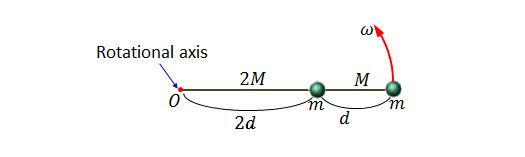Classical Mechanics

# Rotational kinetic energy of extended objectsTwo identical particles each of mass $m=10\text{ kg}$ and two thin rods are in a line, connected to each other as shown in the figure above. The two particles and two rods rotate about the rotational axis $O$ with an angular speed of $\omega=1\text{ rad/s}.$ If the two rods have mass and length of $(2M=4\text{ kg}, 2d=100\text{ cm})$ and $(M=2\text{ kg}, d=50\text{ cm}),$ respectively, what is the (approximate) kinetic energy of the system?

As shown in the figure below, the rotational inertia of a thin rod that rotates about axis through its center perpendicular to length is $\displaystyle I=\frac{1}{12}ML^2,$ where $M$ and $L$ are the mass and length of the thin rod, respectively.What is the approximate rotational inertia of a wheel which has a kinetic energy of $24,600\text{ J}$ and is rotating at $604\text{ rev/min}?$

A uniform solid cylinder is rotating about its central axis at $245\text{ rad/s}.$ The mass and radius of the cylinder are $1.25\text{ kg}$ and $0.50\text{ m},$ respectively. Then what is the approximate rotational kinetic energy of the cylinder?A thin $0.4\text{ kg}$ rod of length $0.70\text{ m}$ hangs from the ceiling, as shown in the figure above. If the rod is pulled to one side and let go, it swings like a pendulum and passes through its lowest position with an angular speed of $4\text{ rad/s}.$ Then what is the rod's approximate kinetic energy at its lowest position? (Neglect friction and air resistance.)

As shown in the figure below, the rotational inertia of a thin rod that rotates about the axis through its center perpendicular to length is $\displaystyle I=\frac{1}{12}ML^2,$ where $M$ and $L$ are the mass and length of the thin rod, respectively.A rod is rotating about the axis which is perpendicular to its length and passes through its center, and two particles are connected to both ends of the rod, as shown in the above figure. The mass of the rod is negligible and the length of the rod is $L=1.00\text{ m},$ and the masses of the two particles are $m_1=12.00\text{ kg}$ and $m_2=11.00\text{ kg}.$ If the angular speed of the system is $4.50\text{ rad/s},$ what is the approximate kinetic energy of the system?

×